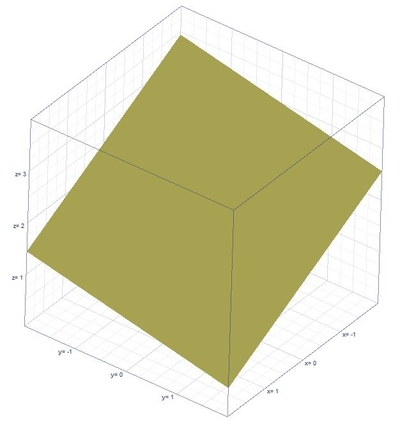# Find the volume of the region of the tetrahedron bounded by the coordinate plane...

## Question:

Find the volume of the region of the tetrahedron bounded by the coordinate plane {eq}\frac{x}{4}+\frac{y}{9}+\frac{z}{2}=1{/eq}. Sketch the tetrahedron in {eq}r^3{/eq}.

## Volume of the Region:

Finding the volume of the region using the double integrals formula which is {eq}V=\int \int zdydx {/eq} where {eq}z {/eq} represents the length and {eq}\int \int dydx {/eq} represents the area.

## Answer and Explanation:

Below is the graph,From the given plane equation,

{eq}z=2-\frac{x}{2}-\frac{2y}{9} {/eq}

When {eq}z=0 {/eq}

{eq}y=9-\frac{9}{4}x {/eq}

When {eq}y=0 {/eq}

{eq}x=4 {/eq}

The volume of the region is,

{eq}V=\int_{0}^{4}\int_{0}^{9-\frac{9}{4}x}zdydx {/eq}

{eq}V=\int_{0}^{4}\int_{0}^{9-\frac{9}{4}x}\left ( 2-\frac{x}{2}-\frac{2y}{9} \right )dydx {/eq}

Integrate with respect to {eq}y {/eq}

{eq}V=\int_{0}^{4}\left [ 2y-\frac{y^2}{9}-\frac{x}{2}y \right ]^{9-\frac{9}{4}x}_{0}dx {/eq}

{eq}V=\int_{0}^{4}\left ( \quad \frac{9x^2}{16}-\frac{9x}{2}+9 \right )dx {/eq}

Integrate with respect to {eq}x {/eq}

{eq}V=\left [ \frac{3x^3}{16}-\frac{9x^2}{4}+9x \right ]^{4}_{0} {/eq}

{eq}V=12 {/eq}

#### Learn more about this topic:Volumes of Shapes: Definition & Examples

from GMAT Prep: Tutoring Solution

Chapter 11 / Lesson 9
45K